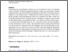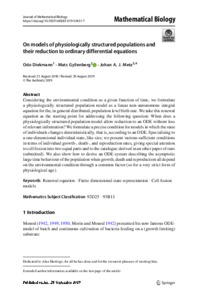# On models of physiologically structured populations and their reduction to ordinary differential equations

Diekmann, O., Gyllenberg, M., & Metz, J. (2019). On models of physiologically structured populations and their reduction to ordinary differential equations. Journal of Mathematical Biology 10.1007/s00285-019-01431-7.Preview
Text
Diekmann2019_Article_OnModelsOfPhysiologicallyStruc.pdf - Published Version

## Abstract

Considering the environmental condition as a given function of time, we formulate a physiologically structured population model as a linear non-autonomous integral equation for the, in general distributed, population level birth rate. We take this renewal equation as the starting point for addressing the following question: When does a physiologically structured population model allow reduction to an ODE without loss of relevant information? We formulate a precise condition for models in which the state of individuals changes deterministically, that is, according to an ODE. Specialising to a one-dimensional individual state, like size, we present various sufficient conditions in terms of individual growth-, death-, and reproduction rates, giving special attention to cell fission into two equal parts and to the catalogue derived in an other paper of ours (submitted). We also show how to derive an ODE system describing the asymptotic large time behaviour of the population when growth, death and reproduction all depend on the environmental condition through a common factor (so for a very strict form of physiological age).

Item Type: Article Renewal equation; Finite dimensional state representation; Cell fission models Evolution and Ecology (EEP) Luke Kirwan 04 Nov 2019 07:25 04 Nov 2019 07:25 http://pure.iiasa.ac.at/16140View Item